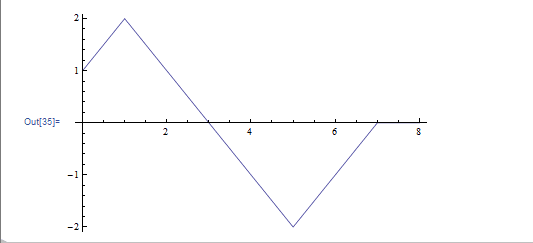# Work done by a variable force question

## Homework StatementThat's a graph of Force in newtons (y axis) vs displacement in meters (x axis) for an object of mass 3.0kg that is moving along the x-axis and initially starts at rest. I am being asked to find the total work done on the object as it moves from x = 0 to x = 7.

## Homework Equations

Net Work = ∫F dx = ΔK
K = 1/2mv^2

## The Attempt at a Solution

So, I integrated the function and ended up with a total work of -.5J. What I want to know is if this violates the work-energy theorem or not. If the net work is equal to change in kinetic energy, and the object starts at rest, this would mean that -.5 = 1/2mv^2. Is there something really obvious that I'm missing here?

work done (energy) = area under F against x graph (this is another way of seeing ΔK = ∫F.dx
There are 2 clear areas to calculate..... when F is +ve and when F is -ve

By calculating the areas I got total work = -0.5J

So, I integrated the function and ended up with a total work of -.5J. What I want to know is if this violates the work-energy theorem or not. If the net work is equal to change in kinetic energy, and the object starts at rest, this would mean that -.5 = 1/2mv^2. Is there something really obvious that I'm missing here?

Can you explain why you think the work-energy theorem is violated?

$0.5=\frac{1}{2}mv^{2}_{f}-\frac{1}{2}mv^{2}_{i}$

If the object started at rest, the last term is 0 and you get

$0.5=\frac{1}{2}mv^{2}_{f}$

which just says that the object has some final velocity.

Can you explain why you think the work-energy theorem is violated?

$0.5=\frac{1}{2}mv^{2}_{f}-\frac{1}{2}mv^{2}_{i}$

If the object started at rest, the last term is 0 and you get

$0.5=\frac{1}{2}mv^{2}_{f}$

which just says that the object has some final velocity.

Right, but if the total work done is -.5, wouldn't that mean that
$-0.5=\frac{1}{2}mv^{2}_{f}$
I'm not sure why you ignored the sign on the work done.